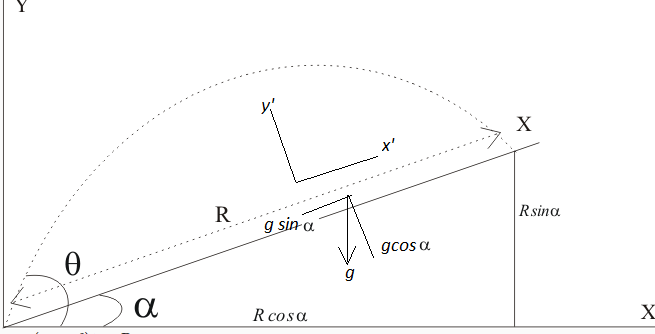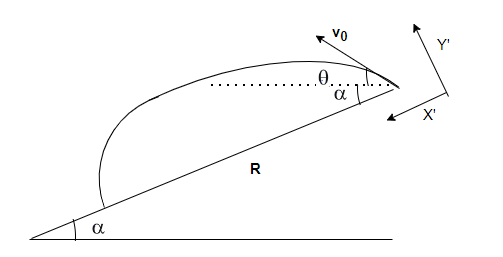# Projectile Motion on a Inclined Plane

## Projectile Motion on a Inclined Plane

• We earliar studied about Projectile motion when ball is thrown up from ground at an angle.Now in this page , we will see Projectile Motion on a Inclined Plane
• Projectile Motion on a Inclined Plane is the motion when a ball or object is thrown at an angle up the inclined plane or down the plane.
• Lets check how to obtain various things like time of flight, range and maximum in each case

## Motion Up The inclined plane• Let the particle be thrown up at an angle $\theta$ from the horizontal on the inclined plane of angle $\alpha$.
• We can obtained the equation of motion using both X-Y axis and X' -Y' plane as shown in the figure. X' -Y' plane will be more convienient
• In X' -Y'
we have
$a_{x'}=-g \sin \alpha$
$a_{y'}=-g \cos \alpha$
$u_{x'}=v_0 cos( \theta - \alpha)$
$u_{y'}=v_0 sin( \theta - \alpha)$
• Now equation of motion along both the x' and y' will be
$x'= v_0 cos( \theta - \alpha) t - \frac {1}{2}g sin \alpha t^2$
$y'= v_0 sin( \theta - \alpha) t - \frac {1}{2}g cos \alpha t^2$
• Now for Time of Flight t=T, we have y'=0,so
$0= v_0 sin( \theta - \alpha) t - \frac {1}{2}g cos \alpha t^2$
or $T = \frac {2 v_0 sin (\theta - \alpha)}{g cos \alpha}$
• So range along the inclined plane will be given by
$x'= v_0 cos( \theta - \alpha) \frac {2 v_0 sin (\theta - \alpha)}{g cos \alpha} - \frac {1}{2}g sin \alpha (\frac {2 v_0 sin (\theta - \alpha)}{g cos \alpha})^2$
$x'=\frac {2v_0^2 sin (\theta - \alpha)}{g cos \alpha}[cos( \theta - \alpha) - \frac {sin \alpha sin (\theta - \alpha)}{cos \alpha}]$
$=\frac {2v_0^2 sin (\theta - \alpha)}{g cos^2 \alpha}[cos \alpha cos( \theta - \alpha) - sin \alpha sin (\theta - \alpha)]$
Now cos(A+B)=(cosAcosB−sinAsinB)
Therefore
$=\frac {2v_0^2 cos \theta sin (\theta - \alpha)}{g cos^2 \alpha}$
Now Applying formula 2 Cos A Sin B=Sin (A+B) - Sin (A-B)
we have
$R=\frac {v_0^2[sin (2\theta -\alpha) - sin \alpha]}{g cos^2 \alpha}$
• In X-Y

we have
$a_{x}=0$
$a_{y}=-g$
$u_{x}=v_0 cos( \theta)$
$u_{y}=v_0 sin( \theta)$
• Now equation of motion along both the x and y will be
$x= v_0 cos( \theta) t$
$y= v_0 sin( \theta) t - \frac {1}{2}g t^2$
• Now when the projectile hit the inclined plane
$x=Rcos \alpha$
$y=R sin \alpha$
• Therefore
$Rcos \alpha= v_0 cos( \theta) t$
$R sin \alpha= v_0 sin( \theta) t - \frac {1}{2}g t^2$
Eliminating t between these two
$R= \frac {2v_0^2 cos^2 \theta}{g cos \alpha}[tan \theta - tan \alpha]$
we can deduce this to same formula
$R=\frac {v_0^2[sin (2\theta -\alpha) - sin \alpha]}{g cos^2 \alpha}$
• Now for Maximum Range, we should $sin (2\theta -\alpha)$ as maximum i.e $sin (2\theta -\alpha)=1$
So Maximum Range will be given as
$R=\frac {v_0^2[sin (2\theta -\alpha) - sin \alpha]}{g cos^2 \alpha}= \frac {v_0^2[1- sin \alpha]}{g cos^2 \alpha}= \frac {v_0^2}{g(1+ sin \alpha)}$

## Motion down The inclined plane• Let the particle be thrown down at an angle $\theta$ from the horizontal on the inclined plane of angle $\alpha$.
• We can obtained the equation of motion using both X-Y axis and X' -Y' plane as shown in the figure. X' -Y' plane will be more convienient
• In X' -Y'
we have
$a_{x'}=-g \sin \alpha$
$a_{y'}=-g \cos \alpha$
$u_{x'}=v_0 cos( \theta + \alpha)$
$u_{y'}=v_0 sin( \theta + \alpha)$
• Now equation of motion along both the x' and y' will be
$x'= v_0 cos( \theta + \alpha) t + \frac {1}{2}g sin \alpha t^2$
$y'= v_0 sin( \theta + \alpha) t - \frac {1}{2}g cos \alpha t^2$
• Now for Time of Flight t=T, we have y'=0,so
$0= v_0 sin( \theta + \alpha) t - \frac {1}{2}g cos \alpha t^2$
or $T = \frac {2 v_0 sin (\theta + \alpha)}{g cos \alpha}$
• So range along the inclined plane will be given by
$R= v_0 cos( \theta + \alpha) T + \frac {1}{2}g sin \alpha T^2$
$=\frac {v_0^2[sin (2\theta +\alpha) + sin \alpha]}{g cos^2 \alpha}$
• Now for Maximum Range, we should $sin (2\theta +\alpha)$ as maximum i.e $sin (2\theta +\alpha)=1$
So Maximum Range will be given as
$R=\frac {v_0^2[sin (2\theta +\alpha) + sin \alpha]}{g cos^2 \alpha}= \frac {v_0^2[1+ sin \alpha]}{g cos^2 \alpha}= \frac {v_0^2}{g(1- sin \alpha)}$

## Solved Examples

Question 1
A football is kicked on a inclined plane of angle 30° at speed 18m/s and angle 60° from the horizontal. Find
1. How much later does it hit the inclined plane?
2.How far from its initial does it hit the incline place?
Solution
Here $v_0=18$ m/s , $\theta =60$ ° and $\alpha =30$°
Time of Flight is given by
$T = \frac {2 v_0 sin (\theta - \alpha)}{g cos \alpha}=\frac {2 \times 18 \times .5}{10 \times .866}=1.16$ sec
Range is given by
$R=\frac {v_0^2[sin (2\theta -\alpha) - sin \alpha]}{g cos^2 \alpha}$=\frac {18 \times 18 \times .5}{10 \times .866 \times .866}=21.60\$ m